# WAVES WAVES WAVES DESCRIBING WAVES Wavelength is the

• Slides: 19WAVES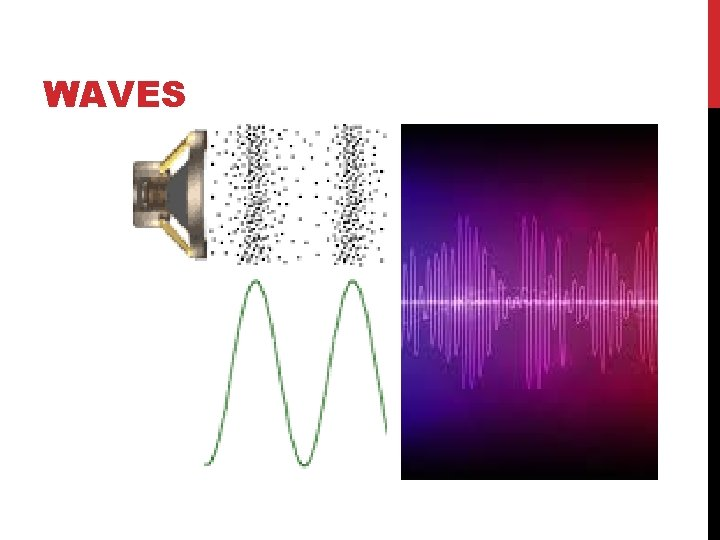WAVESWAVESDESCRIBING WAVES Wavelength (λ) is the distance between a point on one wave and the same point on the next cycle of the wave measured in units λ of length λ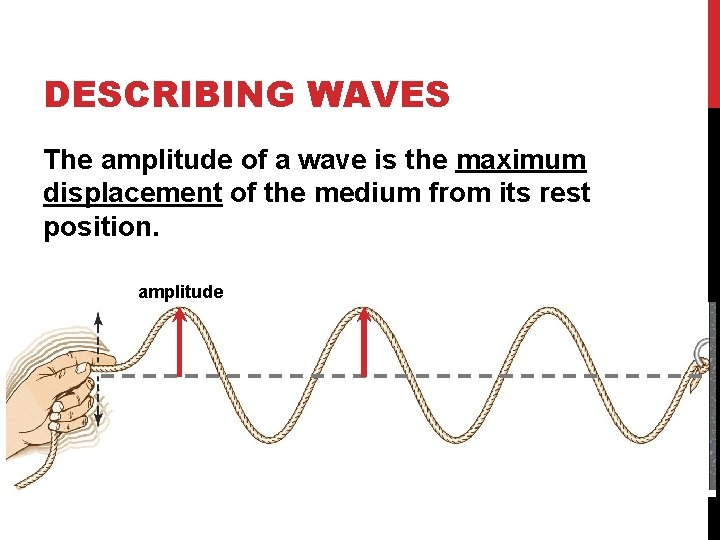DESCRIBING WAVES The amplitude of a wave is the maximum displacement of the medium from its rest position. amplitudePROPERTIES OF WAVES Any motion that repeats at regular time intervals is called periodic motion.PROPERTIES OF WAVES The time required for one cycle, a complete motion that returns to its starting point, is called the period (T). Units?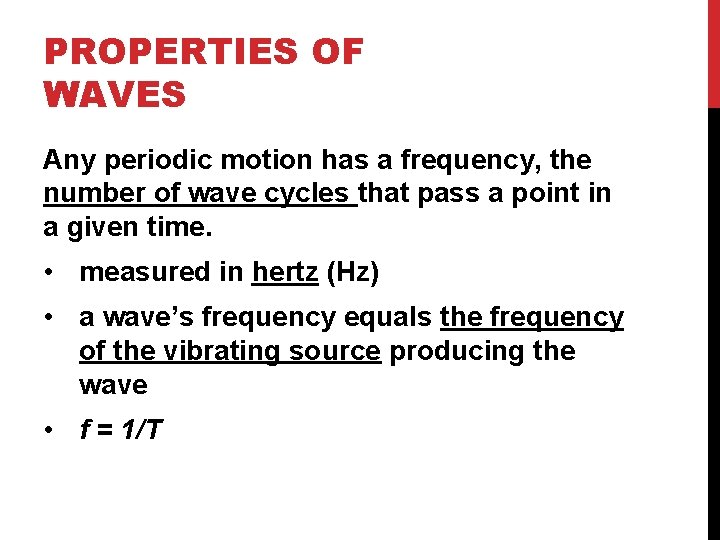PROPERTIES OF WAVES Any periodic motion has a frequency, the number of wave cycles that pass a point in a given time. • measured in hertz (Hz) • a wave’s frequency equals the frequency of the vibrating source producing the wave • f = 1/TFREQUENCY What is the frequency of this wave? How many wave crests pass a certain point each second?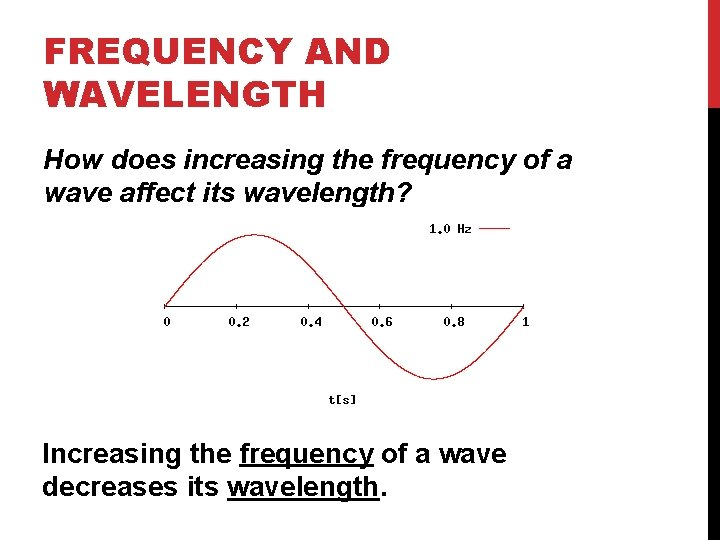FREQUENCY AND WAVELENGTH How does increasing the frequency of a wave affect its wavelength? Increasing the frequency of a wave decreases its wavelength.WAVE SPEED Wave speed is the distance traveled by a given point on the wave (such as a crest) in a given interval of time. You can calculate wave speed by multiplying wavelength by frequency. speed = wavelength x frequency v = fλ Units will be in m/s if wavelength is in m and frequency is in HzAMPLITUDE It takes more energy to produce a wave with higher crests and deeper troughs. The more energy a wave has, the greater is its amplitude. Which wave has the greater energy? Why?GUIDED PRACTICE One end of a rope is vibrated to produce a wave with a wavelength of 0. 25 meters. The frequency of the wave is 3. 0 hertz. What is the speed of the wave? 1. Read and Understand What information are you given? Wavelength = Frequency =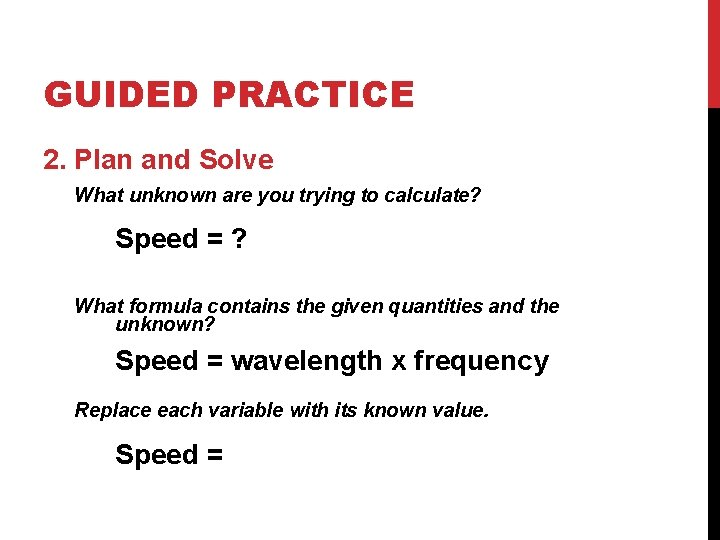GUIDED PRACTICE 2. Plan and Solve What unknown are you trying to calculate? Speed = ? What formula contains the given quantities and the unknown? Speed = wavelength x frequency Replace each variable with its known value. Speed =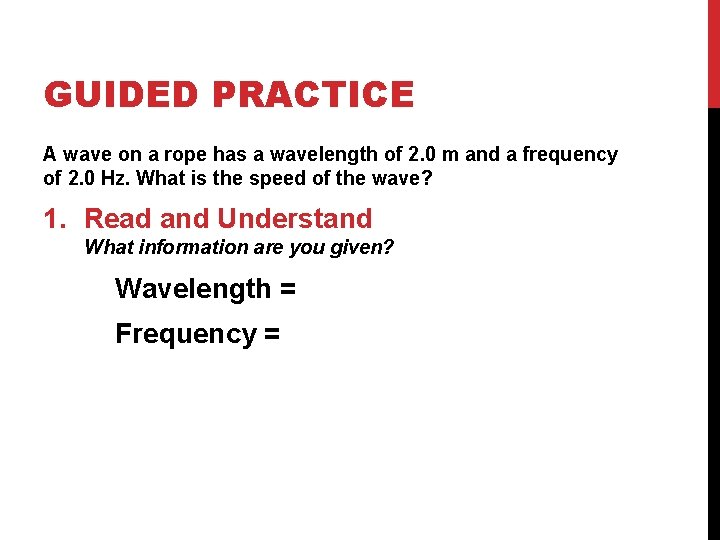GUIDED PRACTICE A wave on a rope has a wavelength of 2. 0 m and a frequency of 2. 0 Hz. What is the speed of the wave? 1. Read and Understand What information are you given? Wavelength = Frequency =GUIDED PRACTICE 2. Plan and Solve What unknown are you trying to calculate? Speed = ? What formula contains the given quantities and the unknown? Speed = wavelength x frequency Replace each variable with its known value. Speed =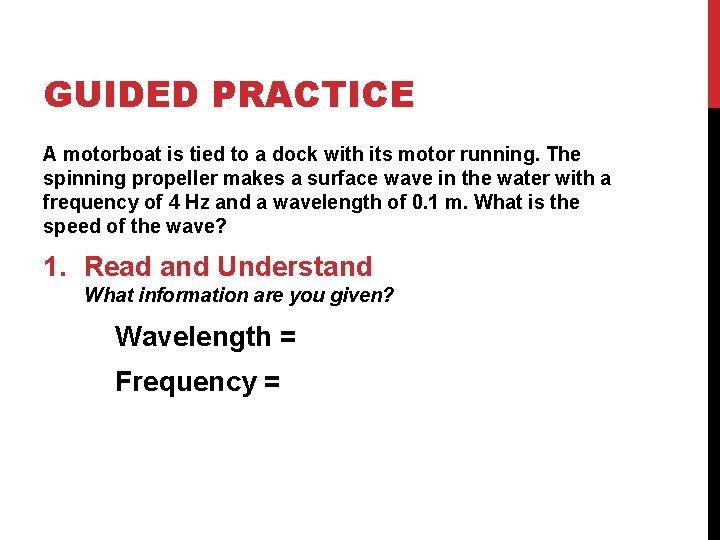GUIDED PRACTICE A motorboat is tied to a dock with its motor running. The spinning propeller makes a surface wave in the water with a frequency of 4 Hz and a wavelength of 0. 1 m. What is the speed of the wave? 1. Read and Understand What information are you given? Wavelength = Frequency =GUIDED PRACTICE 2. Plan and Solve What unknown are you trying to calculate? Speed = ? What formula contains the given quantities and the unknown? Speed = wavelength x frequency Replace each variable with its known value. Speed =INDEPENDENT PRACTICE Silently and independently complete the Wave Problems worksheet, check your answers (taped to front of the room) and turn it in when you’re done.